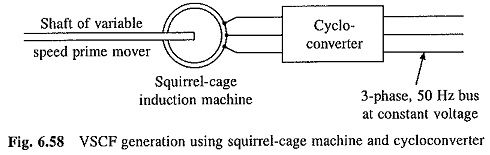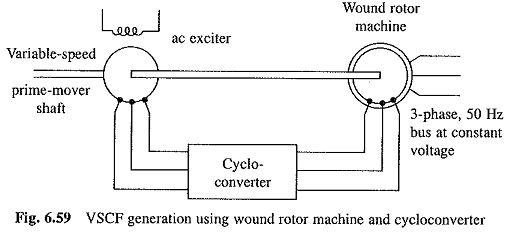## Variable Speed Constant Frequency Generator:

Variable Speed Constant Frequency Generator (VSCF) involves generation of electrical power at fixed frequency and fixed voltage from a variable speed prime mover coupled to the generator shaft. Wind generator is one such example. Speed of rotor varies with velocity and pressure of the wind, but power delivered to the supply mains must be at 50Hz and constant voltage. Of several possible schemes two commonly used are as follows:

### Squirrel Cage Induction Machine and Cycloconverter Scheme:

Squirrel-cage induction motor works as a generator when it is driven at a speed slightly greater than synchronous speed. Lagging reactive power required for establishing flux is obtained from the supply to which it is connected. Further a cycloconverter can couple two sources of different frequency with full four quadrant capability. A VSCF generation scheme using a squirrel-cage generator and cyclo-converter is shwon in Fig. 6.58.As the speed of prime mover varies, frequency of the cycloconverter is continuously changed to ensure that synchronous speed of machine is slightly less than its speed. Lagging reactive power required by the machine is supplied by cycloconverter. Cycloconverter also converts frequency and variable voltage power generated by the machine to power at constant voltage and frequency.

### Wound Rotor Induction Motor and Cycloconverter Scheme (Fig. 6.59):

Rotor of wound-rotor motor is coupled to the shaft of variable speed prime mover. An ac exciter is mounted on the same shaft and feeds its variable frequency output through cycloconverter to the rotor of wound-rotor motor. Cycloconverter controls the frequency and phase sequence of rotor supply such that the speed of field produced by rotor in space remains constant at synchronous speed of the motor for 50 Hz.This ensures that stator generates electrical power at 50 Hz. Cycloconverter can be made to supply the rotor at a constant V/f ratio. Then flux, and therefore, stator output voltage will also be constant.

Scroll to Top